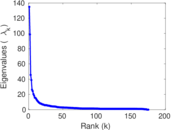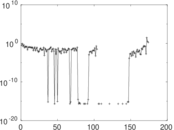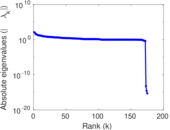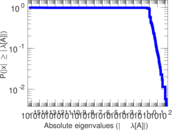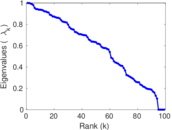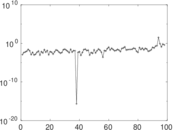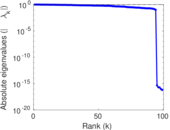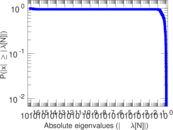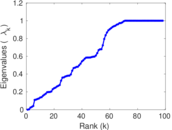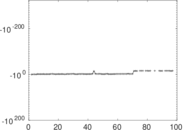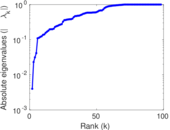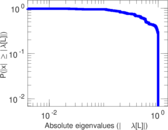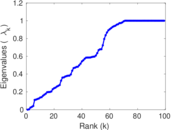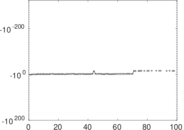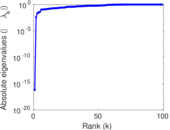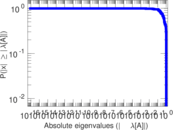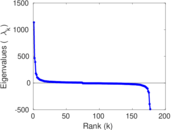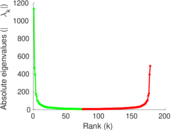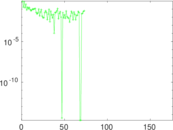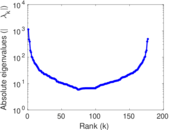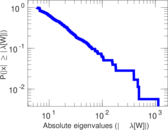Wiktionary edits (om)

This is the bipartite edit network of the Oromo Wiktionary. It contains users and pages from the Oromo Wiktionary, connected by edit events. Each edge represents an edit. The dataset includes the timestamp of each edit.

 Code mom Internal name edit-omwiktionary Name Wiktionary edits (om) Data source http://dumps.wikimedia.org/ AvailabilityDataset is available for download Consistency checkDataset passed all tests Category Authorship network Dataset timestamp 2017-10-20 Node meaning User, article Edge meaning Edit Network formatBipartite, undirected Edge typeUnweighted, multiple edges Temporal dataEdges are annotated with timestamps

Statistics

 Size n = 1,124 Left size n1 = 177 Right size n2 = 947 Volume m = 6,205 Unique edge count m̿ = 2,864 Wedge count s = 242,982 Claw count z = 19,042,409 Cross count x = 1,294,430,294 Square count q = 368,342 4-Tour count T4 = 3,924,700 Maximum degree dmax = 1,318 Maximum left degree d1max = 1,318 Maximum right degree d2max = 56 Average degree d = 11.040 9 Average left degree d1 = 35.056 5 Average right degree d2 = 6.552 27 Fill p = 0.017 086 4 Average edge multiplicity m̃ = 2.166 55 Size of LCC N = 819 Diameter δ = 14 50-Percentile effective diameter δ0.5 = 4.423 73 90-Percentile effective diameter δ0.9 = 7.647 08 Median distance δM = 5 Mean distance δm = 4.849 24 Gini coefficient G = 0.757 696 Balanced inequality ratio P = 0.209 347 Left balanced inequality ratio P1 = 0.099 758 3 Right balanced inequality ratio P2 = 0.242 063 Relative edge distribution entropy Her = 0.803 865 Power law exponent γ = 2.274 80 Tail power law exponent γt = 1.811 00 Tail power law exponent with p γ3 = 1.811 00 p-value p = 0.000 00 Left tail power law exponent with p γ3,1 = 1.651 00 Left p-value p1 = 0.644 000 Right tail power law exponent with p γ3,2 = 8.991 00 Right p-value p2 = 0.069 000 0 Degree assortativity ρ = +0.283 060 Degree assortativity p-value pρ = 6.564 74 × 10−54 Spectral norm α = 134.889 Algebraic connectivity a = 0.003 996 42 Spectral separation |λ1[A] / λ2[A]| = 1.367 03 Controllability C = 759 Relative controllability Cr = 0.688 123

Plots

Fruchterman–Reingold graph drawing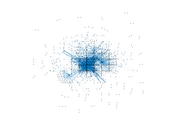Degree distribution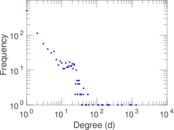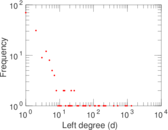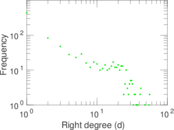Cumulative degree distribution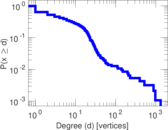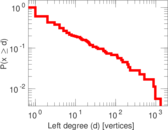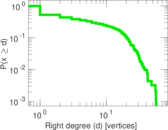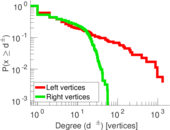Lorenz curve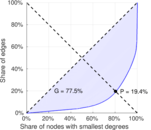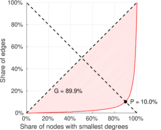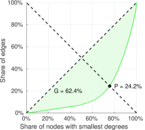Spectral distribution of the adjacency matrix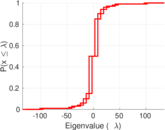Spectral distribution of the normalized adjacency matrix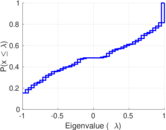Spectral distribution of the Laplacian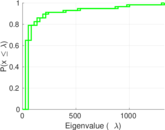Spectral graph drawing based on the adjacency matrix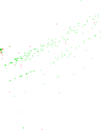Spectral graph drawing based on the LaplacianSpectral graph drawing based on the normalized adjacency matrix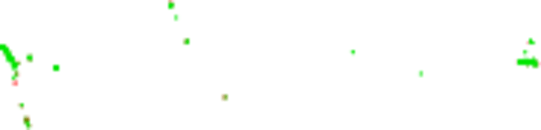Degree assortativity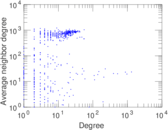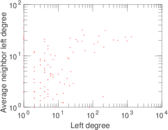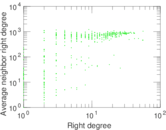Zipf plot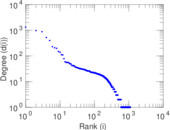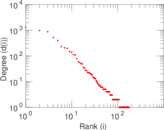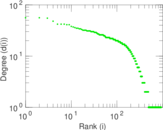Hop distribution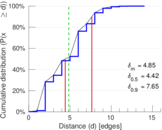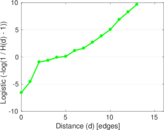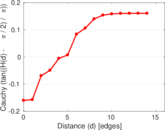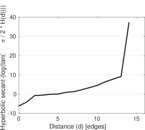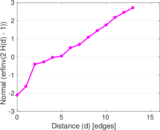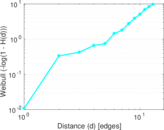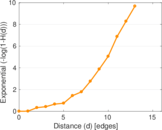Double Laplacian graph drawing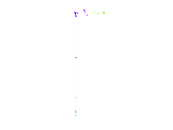Delaunay graph drawing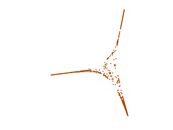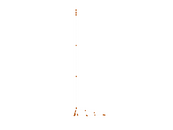Edge weight/multiplicity distribution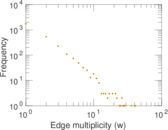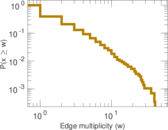Temporal distribution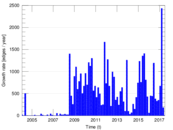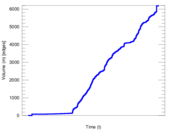Temporal hop distribution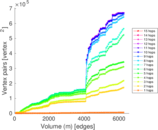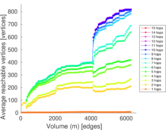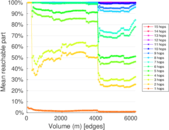Diameter/density evolution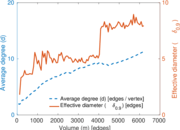Matrix decompositions plots